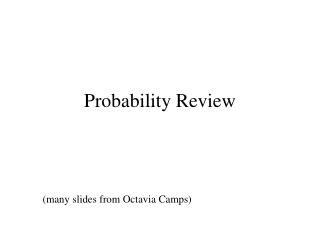DownloadDownload PresentationProbability Review

# Probability Review

Télécharger la présentation## Probability Review

- - - - - - - - - - - - - - - - - - - - - - - - - - - E N D - - - - - - - - - - - - - - - - - - - - - - - - - - -
##### Presentation Transcript

1. Probability Review (many slides from Octavia Camps)

2. Intuitive Development • Intuitively, the probability of an event a could be defined as: Where N(a) is the number that event a happens in n trials

3. More Formal: • W is the Sample Space: • Contains all possible outcomes of an experiment • w2W is a single outcome • A 2W is a set of outcomes of interest

4. Independence • The probability of independent events A, B and C is given by: P(ABC) = P(A)P(B)P(C) A and B are independent, if knowing that A has happened does not say anything about B happening

5. Conditional Probability • One of the most useful concepts! W A B

6. Bayes Theorem • Provides a way to convert a-priori probabilities to a-posteriori probabilities:

7. Using Partitions: • If events Ai are mutually exclusive and partition W W B

8. Random Variables • A (scalar) random variable X is a function that maps the outcome of a random event into real scalar values W X(w) w

9. Random Variables Distributions • Cumulative Probability Distribution (CDF): • Probability Density Function (PDF):

10. Random Distributions: • From the two previous equations:

11. Uniform Distribution • A R.V. X that is uniformly distributed between x1 and x2 has density function: X1 X2

12. Gaussian (Normal) Distribution • A R.V. X that is normally distributed has density function: m

13. Statistical Characterizations • Expectation (Mean Value, First Moment): • Second Moment:

14. Statistical Characterizations • Variance of X: • Standard Deviation of X:

15. Mean Estimation from Samples • Given a set of N samples from a distribution, we can estimate the mean of the distribution by:

16. Variance Estimation from Samples • Given a set of N samples from a distribution, we can estimate the variance of the distribution by:

17. Image Noise Model • Additive noise: • Most commonly used

18. Additive Noise Models • Gaussian • Usually, zero-mean, uncorrelated • Uniform

19. Measuring Noise • Noise Amount: SNR = s/ n • Noise Estimation: • Given a sequence of images I0,I1, … IN-1

20. Good estimators Data values z are random variables A parameter q describes the distribution We have an estimator j (z) of the unknown parameter q. If E(j (z) - q ) = 0or E(j (z) ) = E(q)the estimator j (z) is unbiased

21. Balance between bias and variance Mean squared error as performance criterion

22. Least Squares (LS) If errors only in b Then LS is unbiased But if errors also in A (explanatory variables)

23. Errors in Variable Model

24. Least Squares (LS) bias Larger variance in dA,,ill-conditioned A, u oriented close to the eigenvector of the smallest eigenvalue increase the bias Generally underestimation

25. Estimation of optical flow (a) (b) • Local information determines the component of flow perpendicular to edges • The optical flow as best intersection of the flow constraints is biased.

26. Optical flow • One patch gives a system:

27. Noise model • additive, identically, independently distributed, symmetric noise: